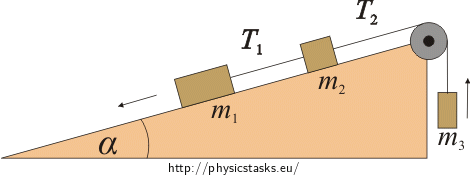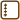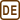## Inclined Plane – Three Bodies

### Task number: 1985

The system depicted in the picture slides down an inclined plane. Determine the acceleration of the system and the magnitudes of the tensile forces T1, T2 acting upon the strings. The coefficient of friction between the blocks and the plane is f. The moment of inertia of the pulley and the mass of the string can be neglected.• #### Given values

 m1 mass of the first weight m2 mass of the second weight m3 mass of the third weight f coefficient of friction α inclination of the plane T1 = ? magnitude of the tensile force between weights m1 and m2 T2 = ? magnitude of the tensile force between weights m2 and m3 a = ? acceleration of the system
• #### Hint 1 – Equations of motion

Draw the picture and highlight the forces acting on each weight. Write down the equations of motion for each weight.

• #### Hint 2 – Friction forces

Realize what the magnitudes of the friction forces depend on and how we can express them.

• #### Hint 3 – Acceleration and magnitudes of the tensile forces

We have a set of 3 equations (8), (9), (10) with three unknowns. By solving this set of equations, we obtain the acceleration and the magnitudes of both tensile forces.

• #### OVERALL SOLUTION

We draw a picture and highlight the forces acting on each weight. We write down the equations of motion for each weight.

Forces acting on the weights:

The weight m1 is acted upon by these forces:

$$\vec{F}_\mathrm{G1}$$…gravitational force

$$\vec{N}_{1}$$…normal force exerted by the plane

$$\vec{T}_{1}$$…tensile force of the string

$$\vec{F}_\mathrm{f1}$$…friction force

The weight m2 is acted upon by these forces:

$$\vec{F}_\mathrm{G2}$$…gravitational force

$$\vec{N}_{2}$$…normal force exerted by the plane

$$\vec{T}_{2}$$…tensile force of the string

$$\vec{T}_{1}\prime$$…tensile force of the string

$$\vec{F}_\mathrm{f2}$$…friction force

The weight m3 is acted upon by these forces:

$$\vec{F}_\mathrm{G2}$$…gravitational force

$$\vec{T}_{2}\prime$$…tensile force of the string

Equations of motion for each weight

All three weights are connected by strings, therefore they move with the same acceleration.

$m_1:\qquad \vec{F}_\mathrm{G1} + \vec{N}_1 + \vec{F}_\mathrm{f1} + \vec{T}_1\,=\,m_1\vec{a},$

$m_2:\qquad \vec{F}_\mathrm{G2} + \vec{N}_2 + \vec{F}_\mathrm{f2} + \vec{T}_1\prime + \vec{T}_2\,=\,m_2\vec{a},$ $m_3:\qquad \vec{F}_\mathrm{G3} + \vec{T}_2\prime\,=\,m_3\vec{a}.$

In order to rewrite the equations of motion using scalars, we choose a coordinate system with axes x, y, such that the x axis follows the direction of the weights motion. The y axis is perpendicular to the x axis.

Forces $$\vec{F}_\mathrm{G_1}$$ a $$\vec{F}_\mathrm{G_2}$$ into two components parallel to these axes:

$F_\mathrm{{G1}_x}\,=\,F_\mathrm{G1}\sin\alpha,$ $F_\mathrm{{G1}_y}\,=\,F_\mathrm{G1}\cos\alpha,$ $F_\mathrm{{G2}_x}\,=\,F_\mathrm{G2}\sin\alpha,$ $F_\mathrm{{G2}_y}\,=\,F_\mathrm{G2}\cos\alpha.$

We rewrite the equations of motion using scalars:

$m_\mathrm{x_1}:\qquad F_\mathrm{G1}sin\alpha - F_\mathrm{f1} - T_1\,=\,m_1a,\tag{1}$ $m_\mathrm{x_2}:\qquad F_\mathrm{G2}sin\alpha - F_\mathrm{f2} + T_1\prime - T_2\,=\,m_2a,\tag{2}$ $m_\mathrm{x_3}:\qquad -F_\mathrm{G3} + T_2\prime\,=\,m_3a,\tag{3}$ $m_\mathrm{y_1}:\qquad N_1 - F_\mathrm{G1} cos\alpha \,=\, 0,\tag{4}$ $m_\mathrm{y_2}:\qquad N_2 - F_\mathrm{G2} cos\alpha \,=\, 0.\tag{5}$

The weights do not move in the direction of the y axis, therefore the acceleration is equal to zero.

Because we neglect the mass of the pulley, it does not have any moment of inertia and does not affect the tensile forces. The weight m3 affects the weight m2 through a string, and conversely the weight m2 affects the weight m3. The situation is similar between weights m1 and m2. According to Newton's third law of motion:

$|\vec{T}_1| \,=\, |\vec{T}_1\prime|,$ $|\vec{T}_2| \,=\, |\vec{T}_2\prime|.$

Friction forces:

A friction force acting upon a weight is directly proportional to the force applied by a weight on the inclined plane. This force is – according to Newton's third law – equal in magnitude to the normal force exerted by the plane on the weight. We can express this relation with an equation:

$F_\mathrm{f1}\,\,=\,\,fN_1,$ $F_\mathrm{f2}\,=\,fN_2.$

Forces N1 and N2 can be expressed from equations (4) and (5):

$N_1\,=\,F_\mathrm{G1}\cos\alpha,$ $N_2\,=\,F_\mathrm{G2}\cos\alpha.$

For the friction forces it holds:

$F_\mathrm{f1}\,=\,fF_\mathrm{G1}\cos\alpha,$ $F_\mathrm{f2}\,=\,fF_\mathrm{G2}\cos\alpha.$

We substitute into the equations of motion (1) and (2):

$m_\mathrm{x_1}:\qquad F_\mathrm{G1}\sin\alpha-fF_\mathrm{G1}\cos\alpha-T_1\,=\,m_1a,\tag{6}$ $m_\mathrm{x_2}:\qquad F_\mathrm{G2}\sin\alpha-fF_\mathrm{G2}\cos\alpha+T_1-T_2\,=\,m_2a.\tag{7}$

We substitute for $$F_\mathrm{{G1}}\,=\,m_1g$$, $$F_\mathrm{{G2}}\,=\,m_2g$$ and $$F_\mathrm{G_3}\,=\,m_3g$$ and rewrite equations (6), (7) and (3):

$m_\mathrm{x_1}:\qquad m_1g\sin\alpha - m_1gf \cos\alpha-T_1\,=\,m_{1}a,\tag{8}$ $m_\mathrm{x_2}:\qquad m_2g\sin\alpha - m_2gf \cos\alpha + T_1 - T_2\,=\,m_2a,\tag{9}$ $m_\mathrm{x_3}:\qquad -m_3g + T_2\,=\,m_3a.\tag{10}$

First, let us calculate the acceleration: a:

By adding equations (8), (9) to equation (10) we get:

$m_1g\sin\alpha-m_1gf\cos\alpha+m_2g\sin\alpha-m_2gf\cos\alpha-m_3g\,=\,$

$\,=\,m_2a+m_3a+m_1a,$ $a\,=\,\frac{m_1g\sin\alpha-m_1gf\cos\alpha+ m_2g\sin\alpha-m_2gf\cos\alpha-m_3g}{m_2+m_3+m_1},$ $a\,=\,\frac{g\left[m_1(\sin\alpha-f\cos\alpha)+ m_2(\sin\alpha-f\cos\alpha)-m_3\right]}{m_2+m_3+m_1},$ $a\,=\,\frac{(m_1+m_2)g(\sin\alpha-f\cos\alpha)-m_3g}{m_2+m_3+m_1}.\tag{11}$

Now, let us calculate the magnitude of the tensile force T1:

We substitute for acceleration from relationship (11) to equation (8):

$m_1g\sin\alpha - m_1gf \cos\alpha-T_1\,=\,$ $\,=\,m_1\frac{(m_1+m_2)g(\sin\alpha-f\cos\alpha)-m_3g}{m_2+m_3+m_1}.$

We express T1 and adjust the expression:

$T_1\,=\,m_1g\sin\alpha-m_1gf \cos\alpha-m_1\frac{(m_1+m_2)g(\sin\alpha-f\cos\alpha)-m_3g}{m_2+m_3+m_1},$

$T_1\,=\,m_1g(\sin\alpha-f\cos\alpha)-\frac{m_1g(m_1+m_2)(\sin\alpha-f\cos\alpha)-m_1m_3g}{m_2+m_3+m_1}.$

We put the expression in terms of a common denominator and adjust it:

$T_1\,=\,\frac{m_1g(\sin\alpha-f\cos\alpha)\left[(m_2+m_3+m_1) -(m_2+m_1)\right]+m_3m_1g}{m_2+m_3+m_1},$ $T_1\,=\,\frac{m_3m_1g\left(\sin\alpha-f\cos\alpha \right)+m_3m_1g}{m_2+m_3+m_1},$ $T_1\,=\,\frac{m_1m_3g(1+\sin\alpha-f\cos\alpha)}{m_2+m_3+m_1}.\tag{12}$

Now, let us calculate the magnitude of the tensile force T2:

We calculateT2 using equation (10):

$T_2\,=\,m_3(a+g).$

We substitute for acceleration from relationship (11):

$T_2\,=\,m_3\left[\frac{(m_1+m_2)g(\sin\alpha-f\cos\alpha)-m_3g} {m_2+m_3+m_1}+g \right].$

We put the expression in terms of a common denominator and adjust it:

$T_2\,=\,m_3\left[\frac{(m_1+m_2)g(\sin\alpha-f\cos\alpha)-m_3g+(m_2+m_1)g+m_3g} {m_2+m_3+m_1}\right],$ $T_2\,=\,\frac{m_3g(m_2+m_1)(1+\sin\alpha-f\cos\alpha)}{m_2+m_3+m_1}.\tag{13}$

The acceleration of the system is

$a\,=\,\frac{(m_1+m_2)g(\sin\alpha-f\cos\alpha)-m_3g}{m_2+m_3+m_1}\,.$

The magnitude of the tensile force between the weight m1 and m2 is

$T_1\,=\,\frac{m_1m_3g(1+\sin\alpha-f\cos\alpha)}{m_2+m_3+m_1}\,.$

The magnitude of the tensile force between the weight m2 and m3 is

$T_2\,=\,\frac{m_3g(m_2+m_1)(1+\sin\alpha-f\cos\alpha)}{m_2+m_3+m_1}\,.$×Original source: Mandíková, D., Rojko, M.: Soubor úloh z mechaniky pro studium učitelství. I. část. Interní materiál, MFF UK, Praha 1994
Zpracováno v bakalářské práci Karolíny Slavíkové (2008).